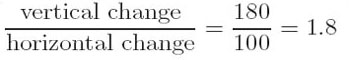First you need some data about corresponding temperatures on each scale. In the case of Celsius and Fahrenheit, there are two fixed points of reference: the freezing and boiling points of water. On the Celsius scale, the freezing point is defined to be 0°C; on the Fahrenheit scale, the freezing point is 32°F. So if you plot degrees Celsius on the horizontal axis and degrees Fahrenheit on the vertical axis of a graph, the freezing point of water is represented by a point with the coordinates
Author(s): The Open University

To determine this, you first need to determine the gradient of the straight line inFigure 11.

The new vertical scale goes from 0 to 180 as the Celsius scale on the horizontal axis goes from 0 to 100. So the gradient isand the relationship be
Author(s): The Open University

Changing the way we work
An introduction to this album.
Author(s): No creator set

In Part B you learned more about the ECHR and the procedures of the ECtHR and how protocols have been used to ensure that the ECHR remains a living instrument. Part B also explored the new challenges created by the rapid expansion of HCPs at the end of the last century and the proposals for reform of the ECtHR.

Author(s): The Open University